# Texas Go Math Grade 3 Lesson 14.5 Answer Key Multiplication Comparisons

Refer to our Texas Go Math Grade 3 Answer Key Pdf to score good marks in the exams. Test yourself by practicing the problems from Texas Go Math Grade 3 Lesson 14.5 Answer Key Multiplication Comparisons.

## Texas Go Math Grade 3 Lesson 14.5 Answer Key Multiplication Comparisons

Unlock the Problem

Carly has 9 pennies. Jack has 4 times as much money as Carly. How can you use multiplication to show how much money Jack has compared to Carly?

Draw a model and write an expression.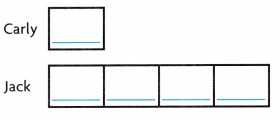Use the model to write an expression.
_______ × ________
Describe the expression as a comparison _______ times as much as _________.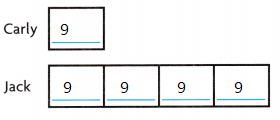9 x 4
Describe the expression as a comparison 4 times as much as 36.

Example

Amelia made 8 cups of lemonade. Nathan made 4 × 8 cups of lemonade. They each wrote a comparison to describe the expression 4 × 8.
Amelia wrote: 4 times as much as 8
Nathan wrote: 4 more than 8
Who described the expression correctly? Explain.
Amelia wrote: 4 times as much as 8
This is the correct statement

Share and Show

Question 1.
There are 8 students in the art club. There are 3 times as many students in chorus. Draw a model and write a multiplication expression to represent the number of students in chorus compared to the art club.Explanation:3 x 8 = 24
3 times as much as 8. is the comparison expression

Math Talk
Mathematical Processes

times
how many times to be multiplied
and for comparison

Draw a model and write a multiplication expression to represent the comparison.

Question 2.
6 times as many as 2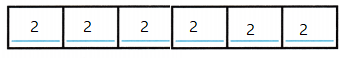Explanation:
6 x 2 = 12

Question 3.
4 times as much as 5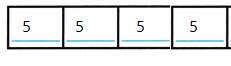Explanation:
4 x 5 = 20

Question 4.
3 times as much as 9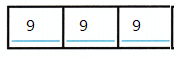Explanation:
3 x 9 = 27

Question 5.
8 times as many as 6
Explanation:
8 x 6 = 48

Describe the multiplication expression as a comparison.

Question 6.
9 × 2
_________ times as many as _________
Explanation:
9 times as many as 2
the multiplication expression as a comparison.

Question 7.
8 × 4
_________ times as much as _________
Explanation:
8 times as much as  4
the multiplication expression as a comparison.

Question 8.
5 × 7
_________ times as much as _________
Explanation:
5 times as much as 7
the multiplication expression as a comparison.

Question 9.
6 × 9
_________ times as many as_________
Explanation:
6 times as much as 9
the multiplication expression as a comparison.

Problem Solving

Question 10.
Use the picture at the right. John’s big dog eats 10 times as much food as his cat eats. Write a multiplication expression to represent the amount of food that John’s dog eats compared to his cat.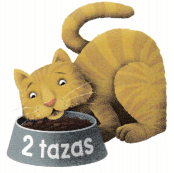2 x 10 = 20
Explanation:
a multiplication expression to represent the amount of food
that John’s dog eats compared to his cat is 2 x 10

Question 11.
H.O.T. Pose a Problem Write a problem about pet food that could be represented using the expression 4 × 3.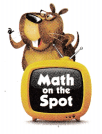Answer: Sims Cow eats Grass 4 times as much food as 3 sheep’s eats
4 x 3
Explanation:
Written a problem about pet food that could be represented using the expression 4 × 3.

Question 12.
H.O.T. Multi-Step Nando has 4 goldfish. Jill has 3 goldfish. Cooper has 2 times as many goldfish as Nando and Jill have combined. Write an expression that compares the number of goldfish that Cooper has with the number of goldfish that Nando and Jill have in all.
Explanation:
Nando and Jill combined is 7$2 times as many as$7
2 x 7= 14
an expression that compares the number of goldfish that Cooper has
with the number of goldfish that Nando and Jill have in all is  2 times as many as \$7

Question 13.
H.O.T. Representations How would the strip diagrams that represent 5 times as much as 11 look different from the strip diagrams that represent 50 times as much as 111
Answer: (50 times as much as 111)10 times as much as (5 times as much as 11 look)

Fill in the bubble for the correct answer choice.

Question 14.
Multi-Step Karen has 2 blue hats, 3 green hats, and 1 red hat. Jonah has 3 times as many hats as Karen. Which expression represents the number of hats that Jonah has compared
(A) 3 × 7
(B) 3 × 6
(C) 6 × 3
(D) 3 + 7
Explanation:
3 × 6 expression represents the number of hats that Jonah has compared

Question 15.
Joseph’s dog weighs 10 pounds. Shaunda’s dog weighs 5 times as many pounds as Joseph’s dog. Which expression represents the weight of Shaunda’s dog compared to Joseph’s dog?
(A) 5 + 10
(B) 10 × 10 × 10 × 10
(C) 10 × 5
(D) 5 × 10
Explanation:
5 × 10 expression represents the weight of Shaunda’s dog compared to Joseph’s dog

Question 16.
Use Math Language Juan has 21 pretzels. Eric’s pretzels can be represented by 6 × 21. Which comparison statement best describes the expression?
(A) Eric has 6 more pretzels than Eric.
(B) Juan has 21 more pretzels than Eric
(C) Eric has 6 times as many pretzels as Juan.
(D) Juan has 6 fewer pretzels than Eric.
Explanation:
Juan has 21 pretzels. Eric’s pretzels can be represented by 6 × 21.
Eric has 6 times as many pretzels as Juan. comparison statement best describes the expression

Texas Test Prep

Question 17.
Use Math Language Which comparison describes the multiplication expression?
8 × 4
(A) 8 more than 4
(B) 4 more than 8
(C) 4 less than 8
(D) 8 times as many as 4
Explanation:
8 times as many as 4 comparison describes the multiplication expression 8 × 4

### Texas Go Math Grade 3 Lesson 14.5 Homework and Practice Answer Key

Draw a model and write a multiplication expression to represent the comparison.

Question 1.
4 times as many as 7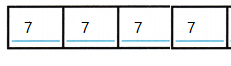Explanation
4 x 7 = 28

Question 2.
5 times as many as 3Explanation:
5 x 3 = 15

Describe the multiplication expression as a comparison.

Question 3.
7 × 6
__________ times as many as __________
Explanation:
7 times as many as 6
the multiplication expression as a comparison.

Question 4.
3 × 8
__________ times as many as __________
Explanation:
3 times as many as 8
the multiplication expression as a comparison.

Question 5.
8 × 5
__________ times as many as __________
Explanation:
8 times as many as 5
the multiplication expression as a comparison.

Question 6.
9 × 2
__________ times as many as __________
Explanation:
9 times as many as 2
the multiplication expression as a comparison.

Problem Solving

Use information in the pictures for 7-9.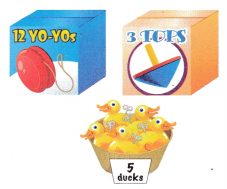Question 7.
The toy store has 4 times as many whistles as yo-yos. Write a multiplication expression to represent the number of whistles compared to yo-yos.
12 x 4 = 48
Explanation:
4 times many as 12
expression to represent the number of whistles compared to yo-yos

Question 8.
Write a comparison statement about the wind-up ducks that could be described using the expression 10 × 5.
10 times as many as 5
Explanation:
Written a comparison statement about the wind-up ducks that could be described using the expression 10 × 5.

Question 9.
The toy store has 8 times as many soccer balls as spinning tops. Write a multiplication expression to represent the number of soccer balls compared to spinning tops.
8 x 3 = 24
Explanation:
multiplication expression to represent the number of soccer balls compared to spinning tops.

Lesson Check

Question 10.
Evelyn sells 5 boxes of greeting cards. Sam sells 3 times as many boxes as Evelyn. Which expression represents the number of boxes that Sam has compared to Evelyn?
(A) 5 × 5 × 5
(B) 5 + 3
(C) 5 – 3
(D) 3 × 5
Explanation:
5 × 5 × 5 expression represents the number of boxes that Sam has compared to Evelyn

Question 11.
Kaitlin collects 6 bottle caps for a prize. Jin collects 4 times as many bottle caps as Kaitlin. Which expression represents the number of bottle caps that Jin collects compared to Kaitlin?
(A) 6 + 4
(B) 6 × 6 × 6 × 6
(C) 4 × 6
(D) 4 × 4 × 4 × 4 × 4 × 4
Explanation:
6 × 6 × 6 × 6 represents the number of bottle caps that Jin collects compared to Kaitlin

Question 12.
Farrah has 18 crayons. John’s crayons can be represented by 2 × 18. Which comparison statement describes the expression?
(A) 18 more than 2
(B) 2 times as many as 18
(C) 2 more than 18
(D) 2 fewer than 18
Explanation:
2 times as many as 18
comparison statement describes the expression

Question 13.
Justin writes the multiplication expression 3 × 9. Which comparison statement describes the expression that Justin wrote?
(A) 3 more than 9
(B) 9 more than 3
(C) 3 times as many as 9
(D) 3 fewer than 9
3 times as many as 9
comparison statement describes the expression that Justin wrote

Question 14.
Multi-Step Bev has 5 books. She buys 3 more books. Dell has 3 times as many books as Bev. Which expression represents the number of books that Dell has compared to Bev?
(A) 3 × 8
(B) 3 × 3
(C) 5 × 8
(D) 3 × 5
Explanation:
5 + 3 = 8
3 x 8 = 24
3 × 8 represents the number of books that Dell has compared to Bev

Question 15.
Multi-Step Ryan has 5 crayons. He gets 4 more. Cindi has 6 times as many crayons as Ryan. Which expression represents the number of crayons that Cindi has compared to Ryan?
(A) 9 + 6
(B) 6 × 9
(C) 4 × 6
(D) 6 × 5